### Type to searchTags:

# LOD UseCase#1 – Finding Monthly Percentage Contribution of Sale to the Total Sales using Calculated Field To Understand LOD

##### Share

LOD: Level of Detail

During my Corporate Tableau Training in Gurgaon , i get questions many time regarding LOD in Tableau .

1. LOD was introduced in Tableau 9

2. LOD facilities tableau users to do advance calculation that combines summaries of Data at different Granularity.

3. We will quickly go through a use case to understand this important concept at its very basic.

For Corporate training and Online Training contact at info@instrovate.com

The Question that we would like to answer to understand this concept and even you can give it a try before you actually see the answer below is “Show a visualization with the Monthly Percentage Contribution of Sale to the Total Sales of that Year”

So what is the challenge here? We want to see the Monthly Percentage Contribution to the Total Sales of that year i.e. Sales in a Particular Month or the Year / Total Sales in that Year

So if you want to see the Monthly Percentage Contribution of September for 2016, the formula would be

= Total Sales in the Month of September 2016 / Total Sales for the Year of 2016.

If we want to see the Total Sales in year (Data Set – Sample Super Store )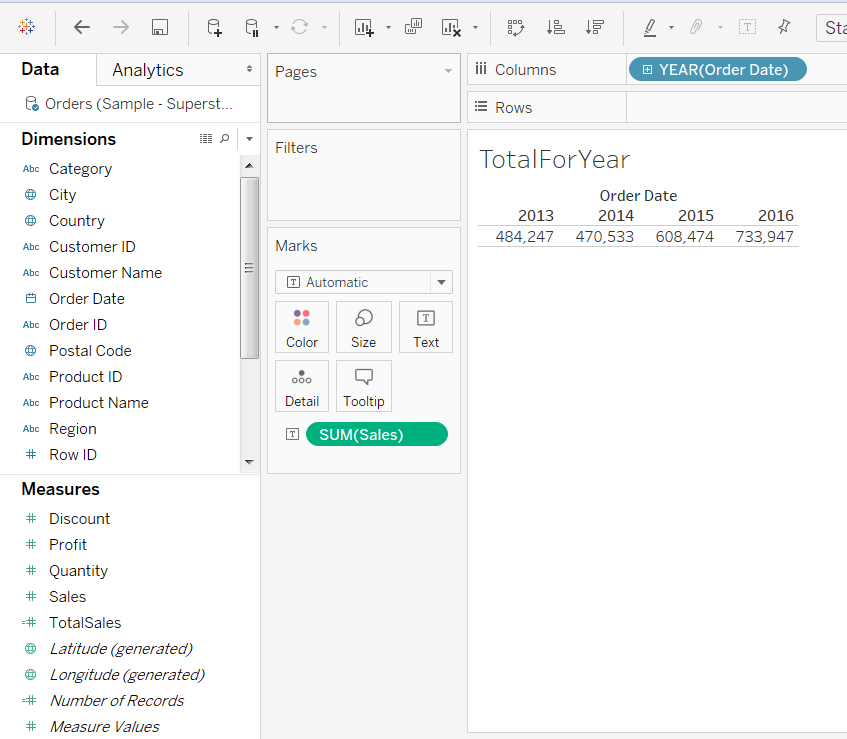If we want to see the Monthly sales for the year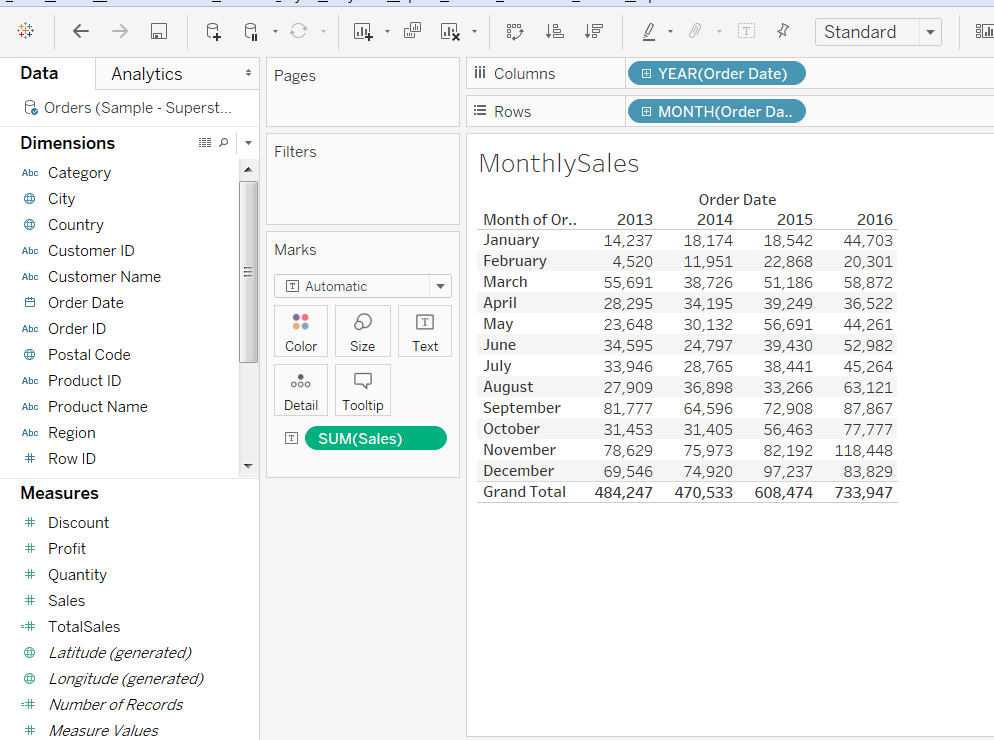Now in the above Visualization, if we want to see percentage sales in each month, it would mean Divide the Sales of that month / Total Sales of the Year, so for September 2016 example it would be

87,867 / 733,947 = 11.97 %

So to do this calculation in tableau, we just need to divide the monthly sales in above view by the total Sales of that year. But before we do that, can you show the Total Sales of Each Year on the Monthly View i.e. as below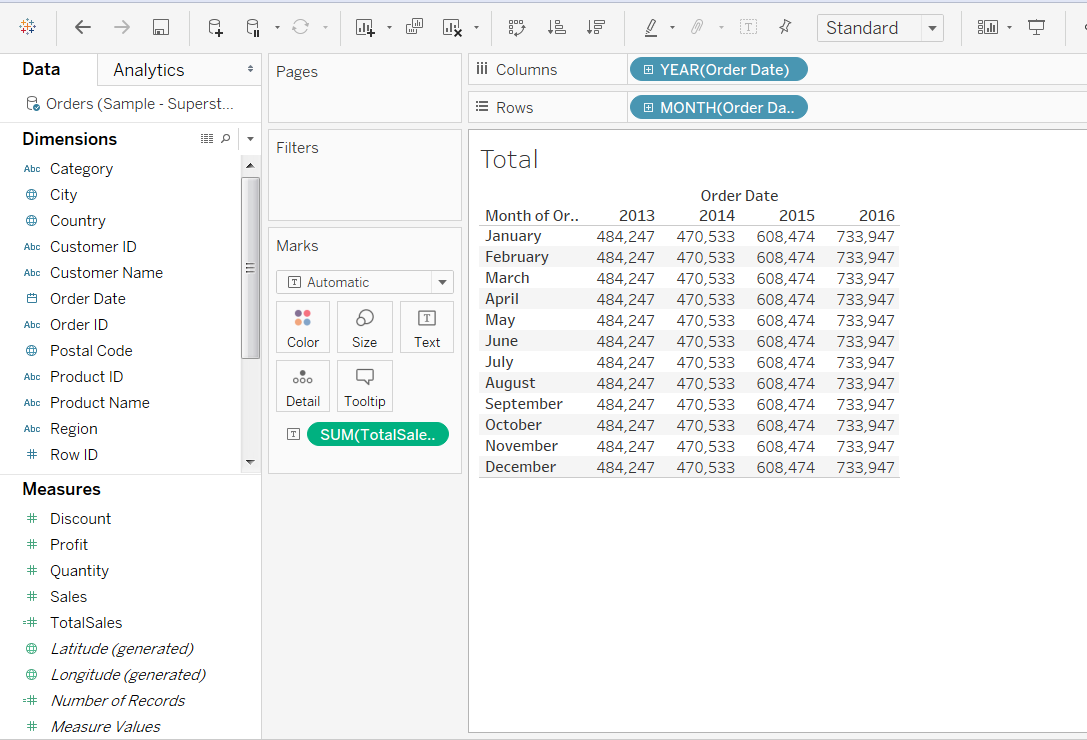If we can get the total sales of the year calculation on monthly basis , it would just be a matter of dividing the monthly sales by this number .

We can do this calculation by using LOD. So in order to get the above visualization we will create a LOD calculate field as “Total Sales “= { FIXED YEAR([Order Date]): SUM([Sales])}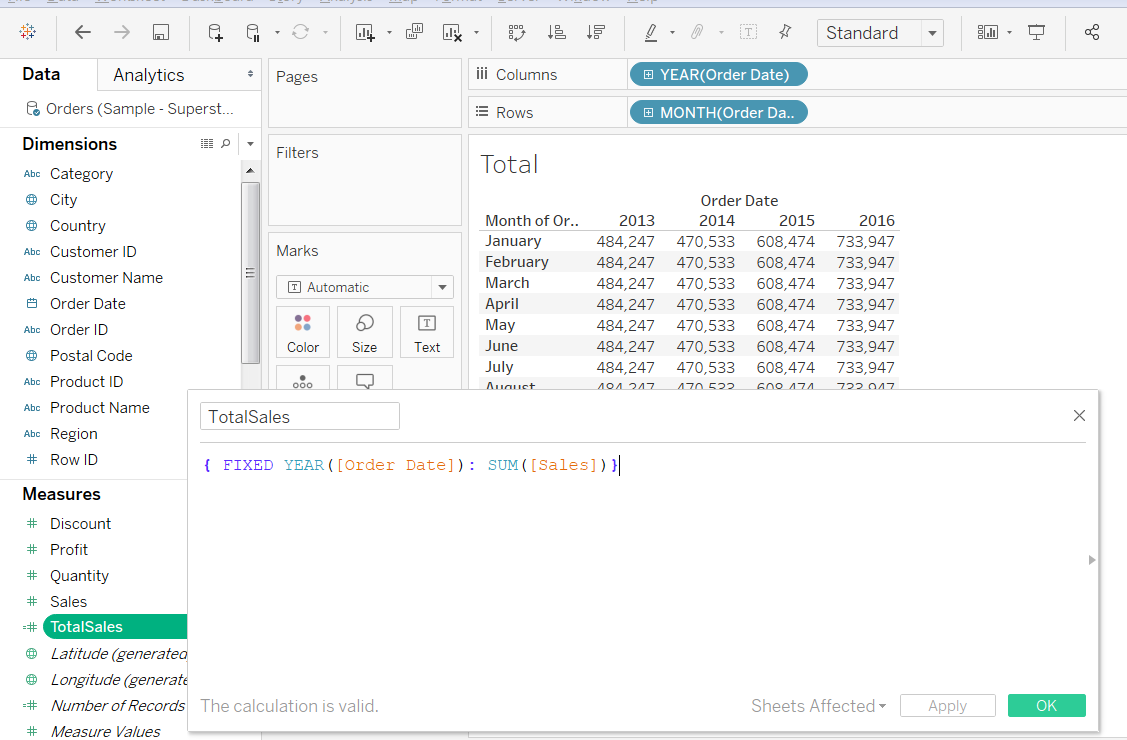In a normal scenario if you select Year , Month and Sales , you get the Sales in each month. If you create a LOD calculated field and fix the value on Yearly basis, the total sum for the year is calculated , even though you are selecting the monthly visualization . This is the beauty of LOD .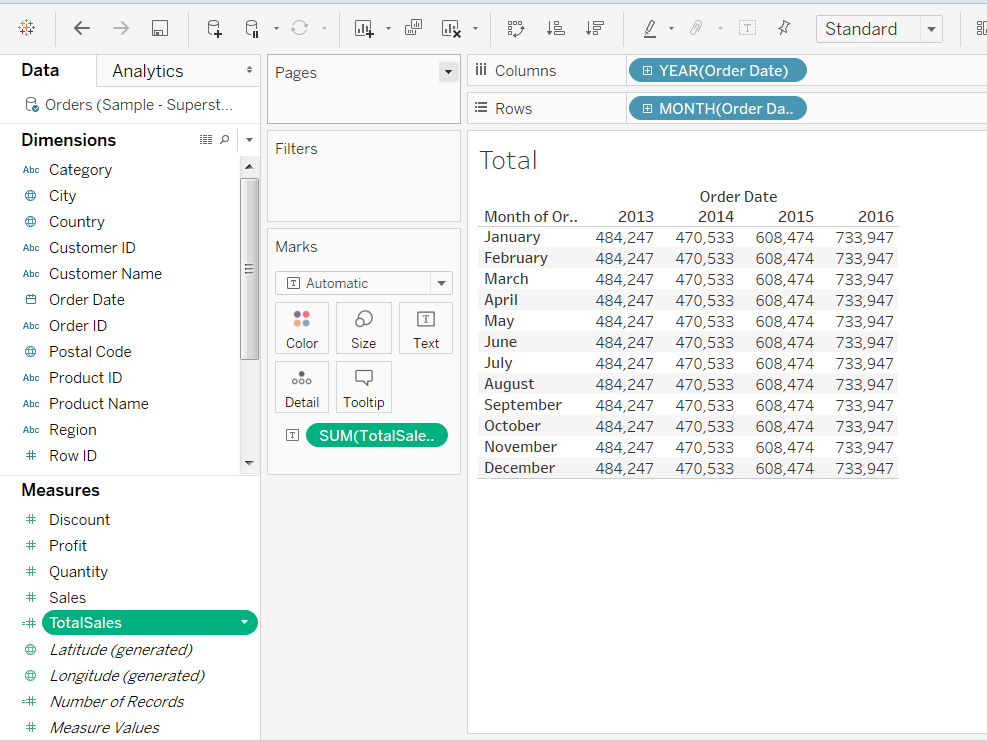Now to get Percentage in each month , do an adhoc calculation i.e. sum(sales) / Sum ( Total Sales) and format this ratio to Percentage.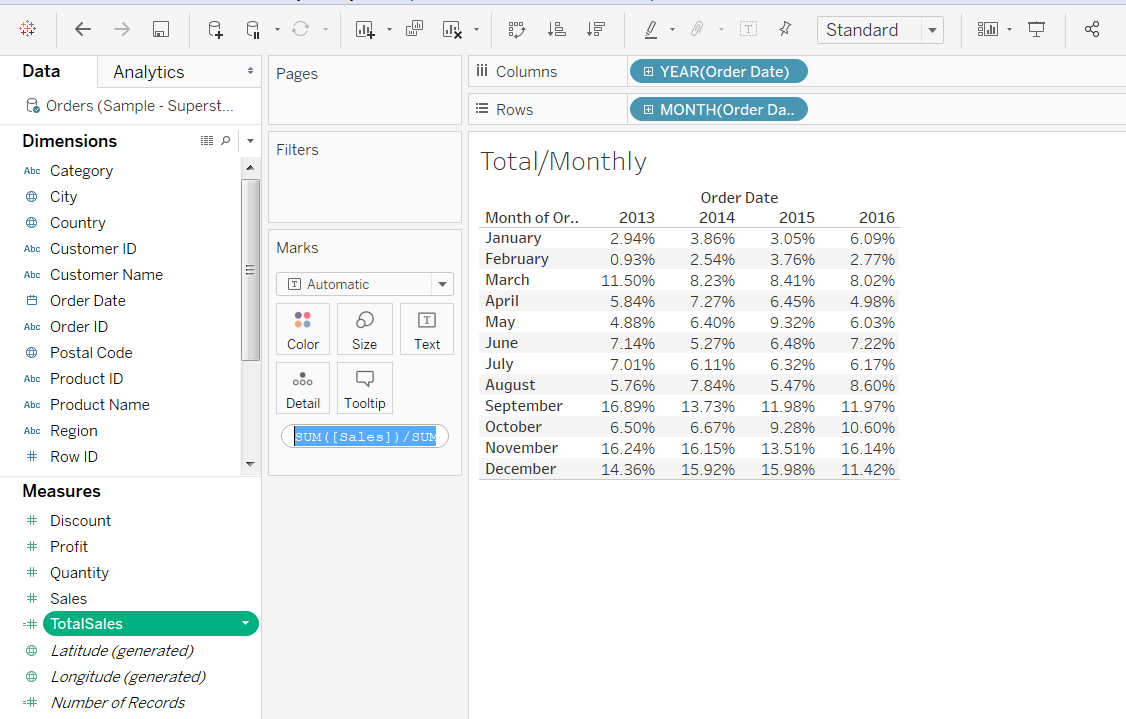Alternatively, what we want to achieve can be easily done by using Table Calculations. Just have the monthly view, drag sales to the visualization and use Table calculations “Percent of Total”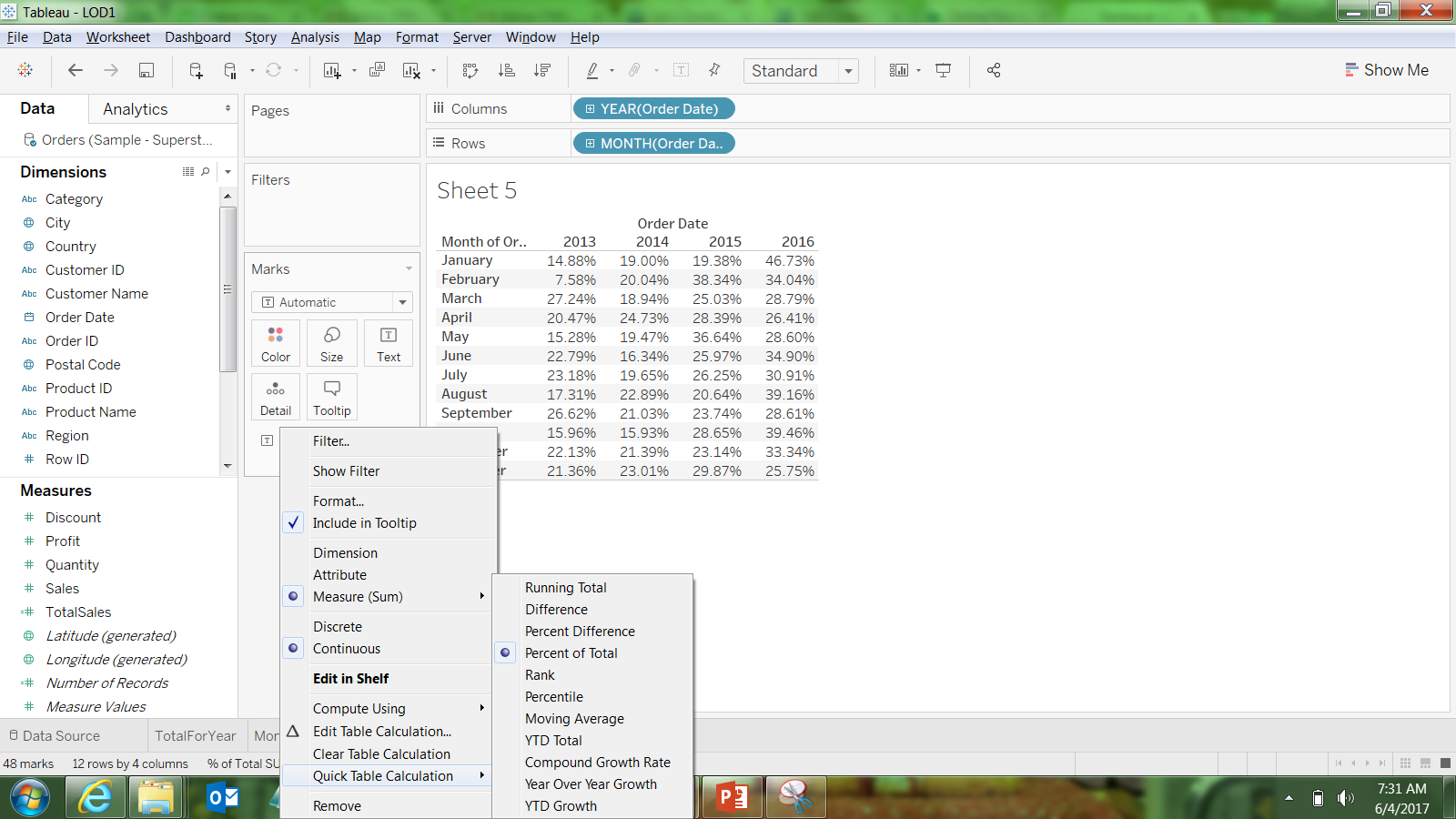And Edit Table Calculation , Compute Using “Table ( down)”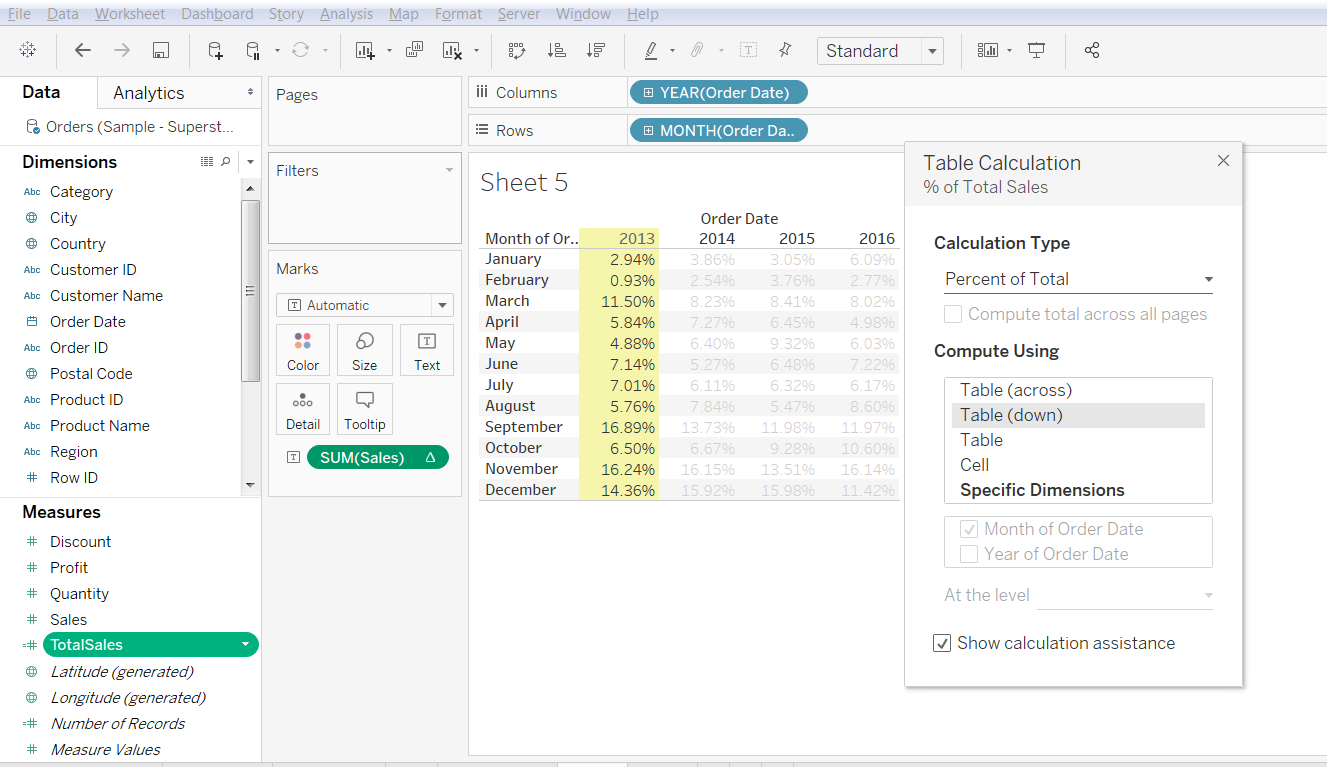So using a Calculated field with LOD to calculate the percentage definately improves your concept of calculation and also how Table Calculation would be working .

For Corporate training and Online Training contact at info@instrovate.com

Tags: